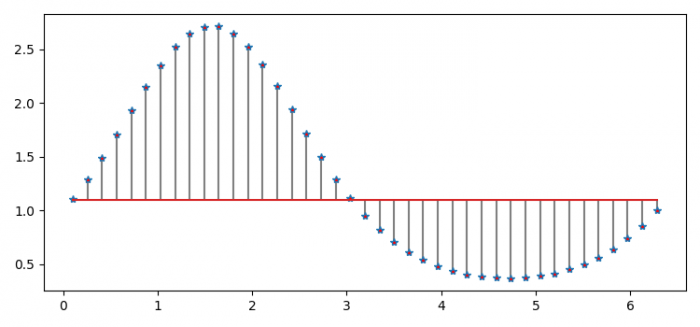# How to a plot stem plot in Matplotlib Python?

To plot a stem plot in Matplotlib, we can use stem() method. It creates vertical lines from a baseline to the Y-coordinate and places a marker at the tip.

## Steps

• Set the figure size and adjust the padding between and around the subplots.
• Create x and y data points using numpy.
• Create a stem plot using stem() method.
• Set the marker face color with red color.
• To display the figure, use show() method.

## Example

import matplotlib.pyplot as plt
import numpy as np

plt.rcParams["figure.figsize"] = [7.50, 3.50]
plt.rcParams["figure.autolayout"] = True

x = np.linspace(0.1, 2 * np.pi, 41)
y = np.exp(np.sin(x))

markerline, stemlines, baseline = plt.stem(x, y, linefmt='grey', markerfmt='*', bottom=1.1)

markerline.set_markerfacecolor('red')

plt.show()

## Output